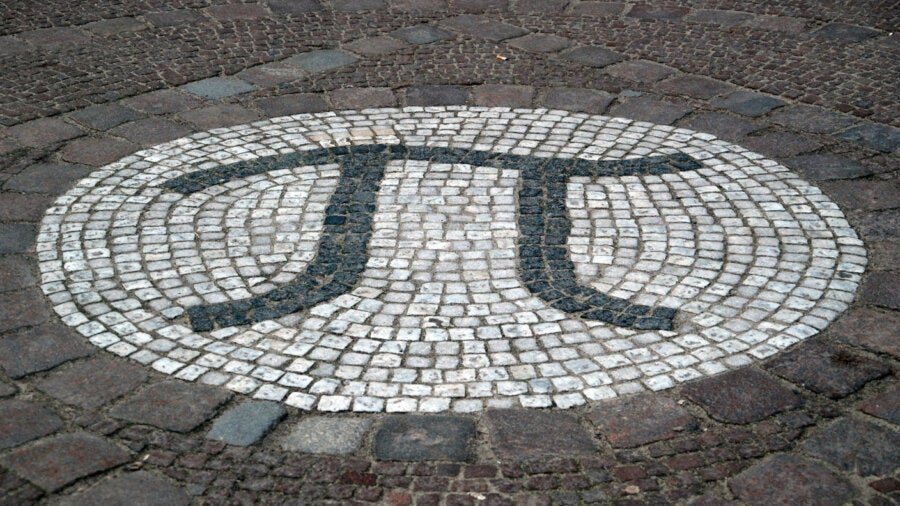# Why Bother Calculating Pi to 62.8 Trillion Digits? It’s Both Useless and FascinatingSwiss researchers at the University of Applied Sciences Graubünden this week claimed a new world record for calculating the number of digits of pi—a staggering 62.8 trillion figures. By my estimate, if these digits were printed out they would fill every book in the British Library ten times over. The researchers’ feat of arithmetic took 108 days and 9 hours to complete, and dwarfs the previous record of 50 trillion figures set in January 2020.

But why do we care?

The mathematical constant pi (π) is the ratio of a circle’s circumference to its diameter, and is approximately 3.1415926536. With only these ten decimal places, we could calculate the circumference of Earth to a precision of less than a millimeter. With 32 decimal places, we could calculate the circumference of our Milky Way galaxy to the precision of the width of a hydrogen atom. And with only 65 decimal places, we would know the size of the observable universe to within a Planck length—the shortest possible measurable distance.

What use, then, are the other 62.79 trillion digits? While the short answer is that they are not scientifically useful at all, mathematicians and computer scientists will be eagerly awaiting the details of this gargantuan computation for a variety of reasons.

### What Makes Pi So Fascinating?

The concept of pi is simple enough for a primary school student to grasp, yet its digits are notoriously difficult to calculate. A number like 1/7 needs infinitely many decimals to write down—0.1428571428571…—but the numbers repeat themselves every six places, making it easy to understand. Pi, on the other hand, is an example of an irrational number, in which there are no repeating patterns. Not only is pi irrational, but it is also transcendental, meaning it cannot be defined through any simple equation featuring whole numbers.

Mathematicians around the world have been computing pi since ancient times, but techniques to do so changed dramatically after the 17th century, with the development of calculus and the techniques of infinite series. For example, the Madhava series (named after the Indian-Hindu mathematician Madhava of Sangamagrama), says:

π = 4(1 – 1/3 + 1/5 – 1/7 + 1/9 – 1/11 + …)

By adding more and more terms, this computation gets closer and closer to the true value of pi. But it takes a long time—after 500,000 terms, it produces only five correct decimal places of pi!

The search for new formulae for pi adds to our mathematical understanding of the number, while also letting mathematicians vie for bragging rights in the quest for more digits. The infinite sum used in the 2020 record-breaking effort was discovered in 1988 and can calculate 14 new digits of pi for each new term that is added to the sum.

While breaking the record may be one of the key motivators for finding new digits of pi, there are two other important benefits.

The first is the development and testing of supercomputers and new high-precision multiplication algorithms. Optimizing the computation of pi leads to computer hardware and software that benefit many other areas of our lives, from accurate weather forecasting to DNA sequencing and even COVID modeling.

The latest computation of pi was 3.5 times as fast as the previous effort, despite the extra 12 trillion decimal places—an impressive increase in supercomputing performance in just 18 months.

The second is the exploration of the very nature of pi. Despite centuries of research, there are still fundamental unanswered questions about the way its digits behave. It is conjectured that pi is a “normal” number, meaning all possible sequences of digits should appear equally often.

For example, we expect the digit 3 to appear as often as the digit 8, and the digit string “12345” to appear as often as “99999.” But we don’t even know if each decimal digit appears infinitely often in pi, let alone whether there are more complex patterns waiting to be discovered.

The data for the new pi computation have not yet been released, as the researchers are awaiting confirmation from the Guinness Book of Records. But we hope there will be many mathematically interesting treasures within the numbers.

We will never “finish” computing the digits of pi—there will always be more to find and new records to break. If you don’t happen to own a supercomputer, but you have a thirst for computing decimal digits (and a PhD in mathematics), why not try other interesting irrational numbers like √3 (only known to 10 billion digits), the tribonacci constant (20,000 digits), or the twin prime constant (1,001 digits). You may not make the morning news, but it’s arguably an easier way to write yourself into the record books.This article is republished from The Conversation under a Creative Commons license. Read the original article.

Image Credit: Holger Motzkau / Wikimedia Commons